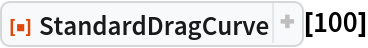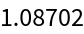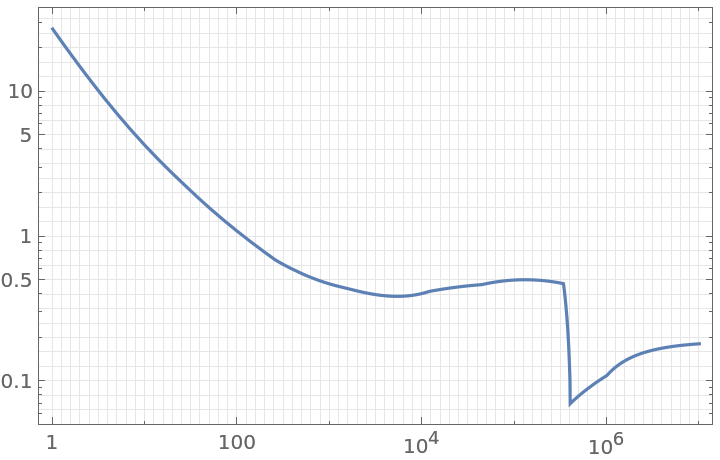Function Repository Resource:

# StandardDragCurve

One of the more popular functions to describe the conventional correlation for the drag on a sphere in steady motion

Contributed by: Björn Zimmermann
 ResourceFunction["StandardDragCurve"][re] gives the drag (force) coefficient on a sphere in steady motion as a function of the Reynolds number re.

## Details and Options

The conventional correlation for the drag on a sphere in steady motion is presented as a graph, called the "standard drag curve", where the drag coefficient CD is plotted as a function of the Reynolds number Re.
Many empirical or semiempirical equations have been proposed to approximate this curve.
Here, a composite of various other range-limited approximations is used.

## Examples

### Basic Examples (2)

Get the drag coefficient for a Reynolds number well in the laminar flow regime:

 In:=Out=Plot the drag (force) coefficient as a function of the Reynolds number. Note the transition to turbulent flow near a Reynolds number of 4×105:

 In:=Out=## Version History

• 1.0.0 – 11 August 2020